# At least three solutions for the Hamiltonian system and reduction method

## Abstract

We investigate the multiplicity of solutions for the Hamiltonian system with some asymptotically linear conditions. We get a theorem which shows the existence of at least three 2π-periodic solutions for the asymptotically linear Hamiltonian system. We obtain this result by the variational reduction method which reduces the infinite dimensional problem to the finite dimensional one. We also use the critical point theory and the variational method.

MSC:35A15, 37K05.

## 1 Introduction and statement of the main result

Let $G\left(t,z\left(t\right)\right)$ be a ${C}^{2}$ function defined on ${R}^{1}×{R}^{2n}$ which is 2π-periodic with respect to the first variable t. In this paper we investigate the number of 2π-periodic solutions of the following Hamiltonian system:

$\begin{array}{r}\stackrel{˙}{p}\left(t\right)=-{G}_{q}\left(t,p\left(t\right),q\left(t\right)\right),\\ \stackrel{˙}{q}\left(t\right)={G}_{p}\left(t,p\left(t\right),q\left(t\right)\right),\end{array}$
(1.1)

where $p,q\in {R}^{n}$, $z=\left(p,q\right)$. Let J be the standard symplectic structure on ${R}^{2n}$, i.e.,

$J=\left(\begin{array}{cc}0& -{I}_{n}\\ {I}_{n}& 0\end{array}\right),$

where ${I}_{n}$ is the $n×n$ identity matrix. Then (1.1) can be rewritten as

$-J\stackrel{˙}{z}={G}_{z}\left(t,z\left(t\right)\right),$
(1.2)

where $\stackrel{˙}{z}=\frac{dz}{dt}$ and ${G}_{z}$ is the gradient of G. We assume that $G\in {C}^{2}\left({R}^{1}×{R}^{2n},{R}^{1}\right)$ satisfies the following asymptotically linear conditions:

(G1) $G\left(t,z\left(t\right)\right)=o\left({|z|}^{2}\right)$ as $|z|\to 0$, $G\left(t,\theta \right)=0$, ${G}_{z}\left(t,\theta \right)=\theta$, where $\theta =\left(0,\dots ,0\right)$.

(G2) There exist constants α, β (without loss of generality, we may assume $\alpha ,\beta \notin Z$) such that

$\alpha I\le {d}_{z}^{2}G\left(t,z\right)\le \beta I\phantom{\rule{1em}{0ex}}\mathrm{\forall }\left(t,z\right)\in {R}^{1}×{R}^{2n}.$

(G3) Let ${j}_{1}$ be an integer within $\left[\alpha ,\beta \right]$ such that

${j}_{1}-1<\alpha <{d}_{z}^{2}G\left(t,0\right)=\underset{|z|\to 0}{lim}\frac{{G}_{z}\left(t,z\right)\cdot z}{{|z|}^{2}}<{j}_{1}.$

(G4) ${lim}_{|z|\to \mathrm{\infty }}\frac{{G}_{z}\left(t,z\right)\cdot z}{{|z|}^{2}}$ exists and there exists ${j}_{2}={j}_{1}+1$ which satisfies

${j}_{1}<{d}_{z}^{2}G\left(t,\mathrm{\infty }\right)=\underset{|z|\to \mathrm{\infty }}{lim}\frac{{G}_{z}\left(t,z\right)\cdot z}{{|z|}^{2}}<\beta <{j}_{2}.$

(G5) G is 2π-periodic with respect to t.

We are looking for the weak solutions of (1.1) with conditions (G1)-(G5). The 2π-periodic weak solution $z=\left(p,q\right)\in E$ of (1.1) satisfies

i.e.,

where E is introduced in Section 2. By Lemma 2.1 in Section 2, the weak solutions of (1.1) coincide with the critical points of the functional

$f\left(z\right)=\frac{1}{2}{\int }_{0}^{2\pi }\left(-J\stackrel{˙}{z}\right)\cdot z\phantom{\rule{0.2em}{0ex}}dt-{\int }_{0}^{2\pi }G\left(t,z\left(t\right)\right)\phantom{\rule{0.2em}{0ex}}dt={\int }_{0}^{2\pi }p\stackrel{˙}{q}\phantom{\rule{0.2em}{0ex}}dt-{\int }_{0}^{2\pi }G\left(t,z\left(t\right)\right)\phantom{\rule{0.2em}{0ex}}dt.$
(1.3)

Several authors  considered the multiplicity of solutions for the Hamiltonian system. Chang proved in  that if $G\in {C}^{2}\left({R}^{1}×{R}^{2n},{R}^{1}\right)$ satisfies conditions (G2), (G5) and the following additional conditions:

(G3)′ Let ${j}_{0}$, ${j}_{0}+1$, … , and ${j}_{1}$ be all integers within $\left[\alpha ,\beta \right]$ (without loss of generality, we may assume $\alpha ,\beta \notin Z$) such that ${j}_{0}-1<\alpha <{j}_{0}<{j}_{1}<\beta <{j}_{1}+1={j}_{2}$. Suppose that there exist $\gamma >0$ and $\tau >0$ such that ${j}_{1}<\gamma <\beta$ and

$G\left(t,z\right)\ge \frac{1}{2}\gamma {\parallel z\parallel }_{{L}^{2}}^{2}-\tau \phantom{\rule{1em}{0ex}}\mathrm{\forall }\left(t,z\right)\in {R}^{1}×{R}^{2n}.$

(G4)′ ${G}_{z}\left(t,\theta \right)=\theta$ and $j\in \left[{j}_{0},{j}_{1}\right)\cap Z$ such that

$jI<{d}_{z}^{2}G\left(t,\theta \right)<\left(j+1\right)I\phantom{\rule{1em}{0ex}}\mathrm{\forall }t\in {R}^{1},$

then (1.1) has at least two nontrivial 2π-periodic weak solutions. Jung and Choi proved in  that if G satisfies the following conditions:

(G1)$G:{R}^{2n}\to R$ is ${C}^{1}$ with $G\left(\theta \right)=0$.

(G2)There exists $h\in N$ such that

$h

(G3)There exists $m\in N$ such that

$h+2m

or

$h-2m-1

(G4)There exists an integer Γ such that $\mathrm{\Gamma }\le \frac{{G}^{\mathrm{\prime }}\left(z\right)\cdot z}{{|z|}^{2}}\le \mathrm{\Gamma }+1$,

then (1.1) has at least m weak solutions, which are geometrically distinct and nonconstant.

Our main result is the following:

Theorem 1.1 Assume that G satisfies conditions (G1)-(G5). Then system (1.1) has at least three 2π-periodic solutions.

Theorem 1.1 will be proved by the finite dimensional reduction method, the critical point theory and the variational method for the perturbed operator ${A}_{ϵ}$. The finite dimensional reduction method combined with the critical point theory and the variational method reduces the critical point results of the functional $I\left(z\right)$ on the infinite dimensional space to those of the corresponding functional $\stackrel{˜}{I}\left(v\right)$ on the finite dimensional subspace.

The outline of this paper is organized as follows. In Section 2, we introduce the Hilbert normed space E, show that the corresponding functional $I\left(z\right)$ of (1.1) is in ${C}^{1}\left(E,R\right)$, Fréchet differentiable and prove the reduction lemma for the perturbed operator ${A}_{ϵ}$. In Section 3, we show that the reduced functional $-\stackrel{˜}{I}\left(v\right)$ satisfies ${\left(P.S.\right)}_{c}$ condition and $v=0$ is the strict local point of minimum of $\stackrel{˜}{I}\left(v\right)$ and prove Theorem 1.1 by the shape of graph of the reduced functional.

## 2 The perturbed operator ${A}_{ϵ}$

Let ${L}^{2}\left(\left[0,2\pi \right],{R}^{2n}\right)$ denote the set of 2n-tuples of the square integrable 2π-periodic functions and choose $z\in {L}^{2}\left(\left[0,2\pi \right],{R}^{2n}\right)$. Then it has a Fourier expansion $z\left(t\right)={\sum }_{k=-\mathrm{\infty }}^{k=+\mathrm{\infty }}{a}_{k}{e}^{ikt}$, with ${a}_{k}=\frac{1}{2\pi }{\int }_{0}^{2\pi }z\left(t\right){e}^{-ikt}\phantom{\rule{0.2em}{0ex}}dt\in {C}^{2n}$, ${a}_{-k}=\overline{{a}_{k}}$ and ${\sum }_{k\in Z}{|{a}_{k}|}^{2}<\mathrm{\infty }$. Let

$A:z\left(t\right)↦-J\stackrel{˙}{z}\left(t\right)$

with the domain

$\begin{array}{rcl}D\left(A\right)& =& \left\{z\left(t\right)\in {H}^{1}\left(\left[0,2\pi \right],{R}^{2n}\right)|z\left(0\right)=z\left(2\pi \right)\right\}\\ =& \left\{z\left(t\right)\in {L}^{2}\left(\left[0,2\pi \right],{R}^{2n}\right)|\sum _{k\in Z}{\left(ϵ+|k|\right)}^{2}{|{a}_{k}|}^{2}<+\mathrm{\infty }\right\},\end{array}$

where ϵ is a positive small number. Then A is a self-adjoint operator. Let $\left\{{M}_{\lambda }\right\}$ be the spectral resolution of A, and let

${P}_{0}={\int }_{\alpha }^{\beta }d{M}_{\lambda },\phantom{\rule{2em}{0ex}}{P}_{+}={\int }_{\beta }^{+\mathrm{\infty }}d{M}_{\lambda },\phantom{\rule{2em}{0ex}}{P}_{-}={\int }_{-\mathrm{\infty }}^{\alpha }d{M}_{\lambda }.$

Let

${L}_{0}={P}_{0}{L}^{2}\left(\left[0,2\pi \right],{R}^{2n}\right),\phantom{\rule{2em}{0ex}}{L}_{+}={P}_{+}{L}^{2}\left(\left[0,2\pi \right],{R}^{2n}\right),\phantom{\rule{2em}{0ex}}{L}_{-}={P}_{-}{L}^{2}\left(\left[0,2\pi \right],{R}^{2n}\right).$

For each $u\in {L}^{2}\left(\left[0,2\pi \right],{R}^{2n}\right)$, we have the composition

$u={u}_{0}+{u}_{+}+{u}_{-},$

where ${u}_{0}\in {L}_{0}$, ${u}_{+}\in {L}_{+}$, ${u}_{-}\in {L}_{-}$. According to A, there exists a small number $ϵ>0$ such that $-ϵ\notin \sigma \left(A\right)$. Let us define the space E as follows:

$E=D\left({|A|}^{\frac{1}{2}}\right)=\left\{z\in {L}^{2}\left(\left[0,2\pi \right],{R}^{2n}\right)|\sum _{k\in Z}\left(ϵ+|k|\right){|{a}_{k}|}^{2}<\mathrm{\infty }\right\}$

with the scalar product

${\left(z,w\right)}_{E}=ϵ{\left(z,w\right)}_{{L}^{2}}+{\left({|A|}^{\frac{1}{2}}z,{|A|}^{\frac{1}{2}}w\right)}_{{L}^{2}}$

and the norm

$\parallel z\parallel ={\left(z,z\right)}_{E}^{\frac{1}{2}}={\left(\sum _{k\in Z}\left(ϵ+|k|\right){|{a}_{k}|}^{2}\right)}^{\frac{1}{2}}.$

The space E endowed with this norm is a real Hilbert space continuously embedded in ${L}^{2}\left(\left[0,2\pi \right],{R}^{2n}\right)$. The scalar product in ${L}^{2}$ naturally extends as the duality pairing between E and ${E}^{\mathrm{\prime }}={W}^{-\frac{1}{2},2}\left(\left[0,2\pi \right],{R}^{2n}\right)$. We note that the operator ${\left(ϵ+|A|\right)}^{-1}$ is a compact linear operator from ${L}^{2}\left(\left[0,2\pi \right],{R}^{2n}\right)$ to E such that

${\left({\left(ϵ+|A|\right)}^{-1}w,z\right)}_{E}={\left(w,z\right)}_{{L}^{2}}.$

Let

${A}_{ϵ}=ϵI+A.$

Let

${E}_{0}={|{A}_{ϵ}|}^{-\frac{1}{2}}{L}_{0},\phantom{\rule{2em}{0ex}}{E}_{+}={|{A}_{ϵ}|}^{-\frac{1}{2}}{L}_{+},\phantom{\rule{2em}{0ex}}{E}_{-}={|{A}_{ϵ}|}^{-\frac{1}{2}}{L}_{-}.$

Then $E={E}_{0}\oplus {E}_{+}\oplus {E}_{-}$ and for $z\in E$, z has the decomposition $z={z}_{0}+{z}_{+}+{z}_{-}\in E$, where

${z}_{0}={|{A}_{ϵ}|}^{-\frac{1}{2}}{u}_{0},\phantom{\rule{2em}{0ex}}{z}_{+}={|{A}_{ϵ}|}^{-\frac{1}{2}}{u}_{+},\phantom{\rule{2em}{0ex}}{z}_{-}={|{A}_{ϵ}|}^{-\frac{1}{2}}{u}_{-}.$

Thus we have

${\parallel {z}_{0}\parallel }_{{E}_{0}}={\parallel {u}_{0}\parallel }_{{L}_{0}},\phantom{\rule{2em}{0ex}}{\parallel {z}_{+}\parallel }_{{E}_{+}}={\parallel {u}_{+}\parallel }_{{L}_{+}},\phantom{\rule{2em}{0ex}}{\parallel {z}_{-}\parallel }_{{E}_{-}}={\parallel {u}_{-}\parallel }_{{L}_{-}}$

and that ${E}_{0}$, ${E}_{+}$, ${E}_{-}$ are isomorphic to ${L}_{0}$, ${L}_{+}$, ${L}_{-}$, respectively. Let us define the functional $f\left(u\right)$ on ${L}^{2}$ as follows:

$f\left(u\right)=\frac{1}{2}\left({\parallel {u}_{+}\parallel }^{2}+{\parallel {M}_{+}{u}_{0}\parallel }^{2}-{\parallel {M}_{-}{u}_{0}\parallel }^{2}-{\parallel {u}_{-}\parallel }^{2}\right)-{\psi }_{ϵ}\left(z\right),$
(2.1)

where ${M}_{+}={\int }_{0}^{\mathrm{\infty }}d{M}_{\lambda }$, ${M}_{-}={\int }_{-\mathrm{\infty }}^{0}d{M}_{\lambda }$ and ${\psi }_{ϵ}\left(z\right)=\psi \left(z\right)+\frac{ϵ}{2}{\parallel z\left(t\right)\parallel }_{{L}^{2}}^{2}$, $\psi \left(z\right)={\int }_{0}^{2\pi }G\left(t,z\left(t\right)\right)\phantom{\rule{0.2em}{0ex}}dt$. Let

$F\left(z\right)={G}_{z}\left(t,z\left(t\right)\right).$

By $G\in {C}^{2}$ and (G2), $\psi \left(z\right)={\int }_{0}^{2\pi }G\left(t,z\left(t\right)\right)\in {C}^{2}\left(E,{R}^{1}\right)$. Let

${F}_{ϵ}\left(z\right)=ϵI+F\left(z\right)=ϵI+{G}_{z}\left(t,z\left(t\right)\right).$

The system (1.1) is equal to

${A}_{ϵ}\left(z\right)={F}_{ϵ}\left(z\right).$
(2.2)

The Euler equation of the functional $f\left(u\right)$ is the system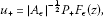(2.3)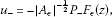(2.4)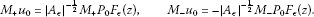(2.5)

Thus $z={z}_{0}+{z}_{+}+{z}_{-}$ is a solution of (2.2) if and only if $u={u}_{0}+{u}_{+}+{u}_{-}$ is a critical point of f. System (2.3)-(2.5) is reduced to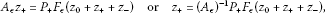(2.6)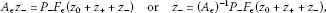(2.7)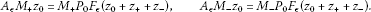(2.8)

By (G2),

${\parallel {F}_{ϵ}\left(u\right)-{F}_{ϵ}\left(v\right)\parallel }_{{L}^{2}}\le \left(ϵ+\beta \right){\parallel u-v\parallel }_{{L}^{2}}\phantom{\rule{1em}{0ex}}\mathrm{\forall }u,v\in {L}^{2}.$
(2.9)

By (G2), there exists a $\gamma >\beta +ϵ$ such that

$\parallel {A}_{ϵ}^{-1}{|}_{{L}_{+}\oplus {L}_{-}}\parallel \le \frac{1}{\gamma }.$

We note that

$f\left(u\right)=f\left(u\left({z}_{0}+{z}_{-}+{z}_{+}\right)\right).$

Let us set

$I\left({z}_{0}+{z}_{+}+{z}_{-}\right)=f\left(u\left({z}_{0}+{z}_{-}+{z}_{+}\right)\right).$

Now we will prove a reduction lemma which reduces the problem on the infinite dimensional space E to that of the finite dimensional subspace.

Let ${z}_{0}\in {E}_{0}$ be fixed and consider the function $h:{E}_{-}×{E}_{+}\to R$ defined by

$h\left({z}_{-},{z}_{+}\right)=I\left({z}_{0}+{z}_{-}+{z}_{+}\right).$

The function h has continuous partial Fréchet derivatives ${D}_{1}h$ and ${D}_{2}h$ with respect to its first and second variables given by

${D}_{i}h\left({z}_{-},{z}_{+}\right)\left({y}_{i}\right)=DI\left({z}_{0}+{z}_{-}+{z}_{+}\right)\left({y}_{i}\right)$
(2.10)

for ${y}_{1}\in {E}_{-}$ and ${y}_{2}\in {E}_{+}$, $i=1,2$. Let $v={z}_{0}$.

Lemma 2.1 Assume that G satisfies the conditions (G1)-(G5).

1. (i)

For given $v\in {E}_{0}$, there exists a unique ${z}_{-}+{z}_{+}\in {C}^{1}\left({E}_{0},{E}_{-}\oplus {E}_{+}\right)$ satisfying the equation

${A}_{ϵ}\left({z}_{-}+{z}_{+}\right)=\left({P}_{-}+{P}_{+}\right){F}_{ϵ}\left(v+{z}_{-}+{z}_{+}\right).$
(2.11)
2. (ii)

There exists ${m}_{1}<0$ such that if ${z}_{-}$ and ${y}_{-}$ are in ${E}_{-}$ and ${z}_{+}\in {E}_{+}$, then

$\left({D}_{1}h\left({z}_{-},{z}_{+}\right)-{D}_{1}h\left({y}_{-},{z}_{+}\right)\right)\left({z}_{-}-{y}_{-}\right)\le {m}_{1}{\parallel {z}_{-}-{y}_{-}\parallel }^{2}.$
(2.12)
3. (iii)

There exists ${m}_{2}>0$ such that if ${z}_{+}$ and ${y}_{+}$ are in ${E}_{+}$ and ${z}_{-}\in {E}_{-}$, then

$\left({D}_{2}h\left({z}_{-},{z}_{+}\right)-{D}_{2}h\left({z}_{-},{y}_{+}\right)\right)\left({z}_{+}-{y}_{+}\right)\ge {m}_{2}{\parallel {z}_{+}-{y}_{+}\parallel }^{2}.$
(2.13)
4. (iv)

For given $v\in {E}_{0}$, if we put the unique solution ${z}_{-}\left(v\right)+{z}_{+}\left(v\right)$ of (2.11) as ${z}_{-}\left(v\right)+{z}_{+}\left(v\right)=\theta \left(v\right)$, then $\theta \left(v\right)$ is continuous on ${E}_{0}$ and satisfies a uniform Lipschitz condition in ${E}_{0}$ with respect to ${L}^{2}$ norm (also norm ${\parallel \cdot \parallel }_{E}$) and ${|{A}_{ϵ}|}^{\frac{1}{2}}{z}_{-}\left(v\right)\in {C}^{1}\left({E}_{0},{E}_{-}\oplus {E}_{+}\right)$, ${|{A}_{ϵ}|}^{\frac{1}{2}}{z}_{+}\left(v\right)\in {C}^{1}\left({E}_{0},{E}_{-}\oplus {E}_{+}\right)$. Moreover,

$DI\left(v+\theta \left(v\right)\right)\left(w\right)=0\phantom{\rule{1em}{0ex}}\mathit{\text{for all}}\phantom{\rule{1em}{0ex}}w\in {E}_{-}\oplus {E}_{+}.$
5. (v)

If $\stackrel{˜}{I}:{E}_{0}\to R$ is defined by

$\stackrel{˜}{I}\left(v\right)=I\left(v+\theta \left(v\right)\right)=I\left(v+{z}_{-}\left(v\right)+{z}_{+}\left(v\right)\right),$

then $\stackrel{˜}{I}$ has a continuous Fréchet derivative $D\stackrel{˜}{I}$ with respect to v, and

$D\stackrel{˜}{I}\left(v\right)\left(y\right)=DI\left(v+\theta \left(v\right)\right)\left(y\right)\phantom{\rule{1em}{0ex}}\mathit{\text{for all}}\phantom{\rule{1em}{0ex}}v,y\in {E}_{0}.$
(2.14)
1. (vi)

$v\in {E}_{0}$ is a critical point of $\stackrel{˜}{I}$ if and only if $v+\theta \left(v\right)=v+{z}_{-}\left(v\right)+{z}_{+}\left(v\right)$ is a critical point of I.

Proof (i) Let $\delta =\frac{\alpha +\beta }{2}+ϵ$. If ${F}_{ϵ}^{\delta }\left(\psi \right)={F}_{ϵ}\left(\psi \right)-\delta$, then equation (2.11) is equivalent to the equation

${z}_{-}+{z}_{+}={\left({A}_{ϵ}-\delta \right)}^{-1}\left({P}_{-}+{P}_{+}\right){F}_{ϵ}^{\delta }\left(v+{z}_{-}+{z}_{+}\right).$
(2.15)

The operator ${\left({A}_{ϵ}-\delta \right)}^{-1}\left({P}_{-}+{P}_{+}\right)$ is a self-adjoint, compact and linear map from $\left({P}_{-}+{P}_{+}\right){L}_{2}$ into itself and its norm is ${\left(min\left\{|{j}_{2}-\delta |,|{j}_{1}-1-\delta |\right\}\right)}^{-1}$. We note that

$\begin{array}{rcl}{\parallel {F}_{ϵ}^{\delta }\left({\psi }_{2}\right)-{F}_{ϵ}^{\delta }\left({\psi }_{1}\right)\parallel }_{{L}^{2}}& \le & \left(max\left\{|\alpha -\delta |,|\beta -\delta |\right\}+ϵ\right){\parallel {\psi }_{2}-{\psi }_{1}\parallel }_{{L}^{2}}\\ =& \left(\frac{\beta -\alpha }{2}+ϵ\right){\parallel {\psi }_{2}-{\psi }_{1}\parallel }_{{L}^{2}}.\end{array}$

We claim that the right-hand side of (2.14) is a Lipschitz mapping of $\left({P}_{-}+{P}_{+}\right){L}_{2}$ into itself with a Lipschitz constant $r<1$. In fact, let v be a fixed element in ${E}_{0}$ and $w=v+{z}_{-}+{z}_{+}$, $y=v+{w}_{-}+{w}_{+}$ be any elements in E. Then we have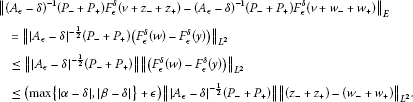Since the operator norm of ${|{A}_{ϵ}-\delta |}^{-\frac{1}{2}}\left({P}_{-}+{P}_{+}\right)$ is less than or equal to $\frac{1}{\sqrt{min\left\{|{j}_{2}-\delta |,|{j}_{1}-1-\delta |\right\}}+ϵ}$ and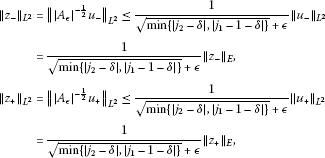we have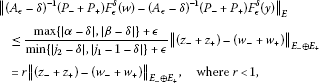since $min\left\{|{j}_{2}-\delta |,|{j}_{1}-1-\delta |\right\}+ϵ>max\left\{|\alpha -\delta |,|\beta -\delta |\right\}+ϵ$. Therefore, by the implicit function theorem, for given $v\in {E}_{0}$, there exists a unique solution ${z}_{-}\left(v\right)+{z}_{+}\left(v\right)\in {E}_{-}\oplus {E}_{+}$ which satisfies (2.15).

1. (ii)

For all ${z}_{-}\in {E}_{-}$,

${\parallel {z}_{-}\parallel }_{E}^{2}\le \left({j}_{1}-1\right){\parallel {w}_{1}\parallel }_{{L}^{2}}^{2}.$
(2.16)

For all ${z}_{+}\in {E}_{+}$,

${\parallel {z}_{+}\parallel }_{E}^{2}\ge \left({j}_{1}+1\right){\parallel {w}_{1}\parallel }_{{L}^{2}\left(\mathrm{\Omega }\right)}^{2}.$
(2.17)

If $v\in {E}_{0}$, ${z}_{-}$ and ${y}_{-}$ are in ${E}_{-}$, ${z}_{+}\in {E}_{+}$ and $z=v+{z}_{-}+{z}_{+}$, then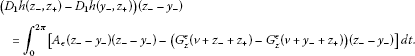Since $\left({G}_{z}^{ϵ}\left({\xi }_{2}\right)-{G}_{z}^{ϵ}\left({\xi }_{1}\right)\right)\left({\xi }_{2}-{\xi }_{1}\right)>\left(\alpha +ϵ\right){\left({\xi }_{2}-{\xi }_{1}\right)}^{2}$ and (2.15) holds, we see that if ${z}_{-}$ and ${y}_{-}$ are in ${E}_{-}$ and ${z}_{+}\in {E}_{+}$, then

$\left({D}_{1}h\left({z}_{-},{z}_{+}\right)-{D}_{1}h\left({y}_{-},{z}_{+}\right)\right)\left({z}_{-}-{y}_{-}\right)\le {m}_{1}{\parallel {z}_{-}-{y}_{-}\parallel }^{2},$

where ${m}_{1}=1-\frac{\alpha }{{j}_{1}-1}<0$.

1. (iii)

Similarly, using the fact that $\left({G}_{z}^{ϵ}\left({\xi }_{2}\right)-{G}_{z}^{ϵ}\left({\xi }_{1}\right)\right)\left({\xi }_{2}-{\xi }_{1}\right)<\left(\beta +ϵ\right){\left({\xi }_{2}-{\xi }_{1}\right)}^{2}$ and (2.17) holds, we see that if ${z}_{+}$ and ${y}_{+}$ are in ${E}_{+}$ and ${z}_{-}\in {E}_{-}$, then

$\left({D}_{2}h\left({z}_{-},{z}_{+}\right)-{D}_{2}h\left({z}_{-},{y}_{+}\right)\right)\left({z}_{+}-{y}_{+}\right)\ge {m}_{2}{\parallel {z}_{+}-{y}_{+}\parallel }^{2},$

where ${m}_{2}=1-\frac{\beta }{{j}_{1}+1}>0$.

1. (iv)

If $\theta \left(v\right)$ denotes the unique $\left({z}_{-}+{z}_{+}\right)\left(v\right)\in {E}_{-}\oplus {E}_{+}$ which solves (2.11), then $\theta \in {C}^{1}\left({E}_{0},E\right)$. In fact, if $v,{v}^{\mathrm{\prime }}\in {E}_{0}$, and ${p}_{1}=\theta \left(v\right)$, ${p}_{2}=\theta \left({v}^{\mathrm{\prime }}\right)$, then we have

$\begin{array}{rcl}{\parallel {p}_{1}-{p}_{2}\parallel }_{E}& =& {\parallel {\left({A}_{ϵ}\right)}^{-1}\left({P}_{-}+{P}_{+}\right)\left[{F}_{ϵ}\left(v+{p}_{1}\right)-{F}_{ϵ}\left({v}^{\mathrm{\prime }}+{p}_{2}\right)\right]\parallel }_{E}\\ \le & C{\parallel \left(v+{p}_{1}\right)-\left({v}^{\mathrm{\prime }}+{p}_{2}\right)\parallel }_{E}\\ \le & C{\parallel \left(v-{v}^{\mathrm{\prime }}\right)-\left({p}_{1}-{p}_{2}\right)\parallel }_{E}.\end{array}$

Thus we have

${\parallel {p}_{1}-{p}_{2}\parallel }_{E}\le \frac{C}{1-C}{\parallel v-{v}^{\mathrm{\prime }}\parallel }_{E}.$

Thus θ is continuous. Since ${F}_{ϵ}\in {C}^{1}\left(E,E\right)$, $\theta \in {C}^{1}\left({E}_{0},E\right)$. Since $dim{L}_{0}$ is finite and all topologies on ${L}_{0}$ are equivalent, we have

${|{A}_{ϵ}|}^{\frac{1}{2}}{z}_{+}\left(v\right)\in {C}^{1}\left({L}_{0},L\right).$

Let $v\in {E}_{0}$. If $q\in {E}_{-}\oplus {E}_{+}$, then from (2.11) we have

${\int }_{0}^{2\pi }\left[{A}_{ϵ}\left(\theta \left(v\right)\right)\cdot q-\left({P}_{-}+{P}_{+}\right){F}_{ϵ}\left(v+\theta \left(v\right)\right)\cdot q\right]\phantom{\rule{0.2em}{0ex}}dt=0.$

Since ${\int }_{0}^{2\pi }{A}_{ϵ}v\cdot q=0$, we have

$DI\left(v+\theta \left(v\right)\right)\left(w\right)={\int }_{0}^{2\pi }\left[{A}_{ϵ}\left(v+\theta \left(v\right)\right)\cdot q-\left({P}_{-}+{P}_{+}\right){F}_{ϵ}\left(v+\theta \left(v\right)\right)\cdot q\right]\phantom{\rule{0.2em}{0ex}}dt=0$

for all $q\in {E}_{-}\oplus {E}_{+}$.

1. (v)

Since the functional I has a continuous Fréchet derivative DI, $\stackrel{˜}{I}$ has a continuous Fréchet derivative $D\stackrel{˜}{I}$ with respect to v.

2. (vi)

Suppose that there exists $v\in {E}_{0}$ such that $D\stackrel{˜}{I}\left(v\right)=0$. From $D\stackrel{˜}{I}\left(v\right)\left(h\right)=DI\left(v+\theta \left(v\right)\right)\left(h\right)$ for all $v,h\in {E}_{0}$, $DI\left(v+\theta \left(v\right)\right)\left(h\right)=0$ for all $h\in {E}_{0}$. Since $DI\left(v+\theta \left(v\right)\right)\left(w\right)$ for all $w\in {E}_{-}\oplus {E}_{+}$, it follows that $DI\left(v+\theta \left(v\right)\right)=0$. Thus $v+\theta \left(v\right)$ is a solution of (1.1). Conversely if u is a solution of (1.1) and $v={P}_{0}u$, then $D\stackrel{˜}{I}\left(v\right)=0$. □

## 3 Proof of Theorem 1.1

Lemma 3.1 Assume that G satisfies the conditions (G1)-(G5). Then $-\stackrel{˜}{I}\left(v\right)$ is bounded below and satisfies $\left(P.S.\right)$ condition.

Proof Let $v\in {E}_{0}$. By the finite dimensional reduction,

$\stackrel{˜}{I}\left(v\right)=\frac{1}{2}\left({A}_{ϵ}\left(v+\theta \left(v\right)\right),v+\theta \left(v\right)\right)-{\int }_{0}^{2\pi }{G}^{ϵ}\left(t,v\left(t\right)+\theta \left(v\left(t\right)\right)\right)\phantom{\rule{0.2em}{0ex}}dt,$

where $\theta \left(v\right)={\theta }_{-}\left(v\right)+{\theta }_{+}\left(v\right)$, $v\in {E}_{0}$, ${\theta }_{-}\left(v\right)\in {E}_{-}$, ${\theta }_{+}\left(v\right)\in {E}_{+}$, ${G}^{ϵ}\left(t,v\left(t\right)+\theta \left(v\left(t\right)\right)\right)=G\left(t,v\left(t\right)+\theta \left(v\left(t\right)\right)\right)+ϵ{\left(v\left(t\right)+\theta \left(v\left(t\right)\right)\right)}^{2}$. Let $w=v+{\theta }_{-}\left(v\right)$. Then we have

$\begin{array}{rcl}\stackrel{˜}{I}\left(v\right)& =& \frac{1}{2}\left({A}_{ϵ}\left(w\right),w\right)-{\int }_{0}^{2\pi }{G}^{ϵ}\left(t,w\left(t\right)\right)\phantom{\rule{0.2em}{0ex}}dt\\ +\left[\frac{1}{2}\left(\left({A}_{ϵ}\left(\theta \left(v\right)\right),\theta \left(v\right)\right)-\left({A}_{ϵ}\left(w\right),w\right)\right)-{\int }_{0}^{2\pi }\left({G}^{ϵ}\left(t,\theta \left(v\left(t\right)\right)\right)-{G}^{ϵ}\left(t,w\left(t\right)\right)\right)\phantom{\rule{0.2em}{0ex}}dt\right].\end{array}$

Moreover, we have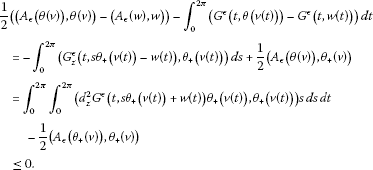By (G4), we have chosen a number γ such that ${j}_{1}<\gamma <{d}_{z}^{2}G\left(t,\mathrm{\infty }\right)<\beta$. Thus we have

Thus $-\stackrel{˜}{I}\left(v\right)$ is bounded from below and satisfies $\left(P.S.\right)$ condition. □

Lemma 3.2 Assume that G satisfies conditions (G1)-(G5). Then $v=0$ is a strict local point of minimum of $\stackrel{˜}{I}\left(v\right)$ with $\stackrel{˜}{I}\left(0\right)=0$.

Proof

$\begin{array}{rcl}\stackrel{˜}{I}\left(v\right)& =& I\left(v+\theta \left(v\right)\right)\\ =& \frac{1}{2}\left({A}_{ϵ}\left(v+\theta \left(v\right)\right),v+\theta \left(v\right)\right)-{\int }_{0}^{2\pi }{G}^{ϵ}\left(t,v\left(t\right)+\theta \left(v\left(t\right)\right)\right)\phantom{\rule{0.2em}{0ex}}dt\\ =& \frac{1}{2}\left({A}_{ϵ}\left(v\right),v\right)+C,\end{array}$

whereThus we have

$\underset{|v|\to 0}{lim}\stackrel{˜}{I}\left(v\right)-\stackrel{˜}{I}\left(0\right)=\frac{1}{2}\left({j}_{1}-{d}_{z}^{2}G\left(t,0\right)\right){\parallel v\parallel }_{{L}^{2}}>0.$

Thus $v=0$ is a strict local point of minimum of $\stackrel{˜}{I}\left(v\right)$. Since $\theta \left(0\right)=0$, $\stackrel{˜}{I}\left(0\right)=0$. □

Proof of Theorem 1.1 By Lemma 2.1(v), $\stackrel{˜}{I}\left(v\right)$ is continuous and Fréchet differentiable in ${E}_{0}$. By Lemma 3.1, $\stackrel{˜}{I}\left(v\right)$ is bounded above, satisfies the $\left(P.S.\right)$ condition and $\stackrel{˜}{I}\left(v\right)\to -\mathrm{\infty }$ as ${\parallel v\parallel }_{E}\to \mathrm{\infty }$. By Lemma 3.2, $v=0$ is a strict local point of minimum of $\stackrel{˜}{I}\left(v\right)$ with a critical value $\stackrel{˜}{I}\left(0\right)=0$. We note that ${max}_{v\in {E}_{0}}\stackrel{˜}{I}\left(v\right)>0$ is another critical value of $\stackrel{˜}{I}$. By the shape of the graph of the functional $\stackrel{˜}{I}$ on the one-dimensional subspace ${E}_{0}$, there exists the third critical point of $\stackrel{˜}{I}\left(v\right)$. Thus (1.1) has at least three solutions, one of which is a trivial solution $u=v+\theta \left(v\right)=0+0=0$. □

## References

1. Chang KC: Infinite Dimensional Morse Theory and Multiple Solution Problems. Birkhäuser, Boston; 1993.

2. Jung T, Choi QH: On the number of the periodic solutions of the nonlinear Hamiltonian system. Nonlinear Anal. TMA 2009, 71(12):e1100-e1108. 10.1016/j.na.2009.01.095

3. Jung T, Choi QH: Periodic solutions for the nonlinear Hamiltonian systems. Korean J. Math. 2009, 17(3):331–340.

4. Rabinowitz PH CBMS Regional Conf. Ser. Math. 65. In Minimax Methods in Critical Point Theory with Applications to Differential Equations. Am. Math. Soc., Providence; 1986.

## Acknowledgements

The authors appreciate very much the referees for their kind corrections. This work (Tacksun Jung) was supported by Basic Science Research Program through the National Research Foundation of Korea (NRF) funded by the Ministry of Education, Science and Technology (KRF-2011-0026920).

## Author information

Authors

### Corresponding author

Correspondence to Q-Heung Choi.

### Competing interests

The authors did not provide this information.

### Authors’ contributions

The authors did not provide this information.

## Rights and permissions

Reprints and Permissions

Jung, T., Choi, QH. At least three solutions for the Hamiltonian system and reduction method. J Inequal Appl 2013, 91 (2013). https://doi.org/10.1186/1029-242X-2013-91

• Accepted:

• Published:

• DOI: https://doi.org/10.1186/1029-242X-2013-91

### Keywords

• Hamiltonian system
• asymptotical linearity
• variational reduction method
• critical point theory
• variational method
• ${\left(P.S.\right)}_{c}$ condition